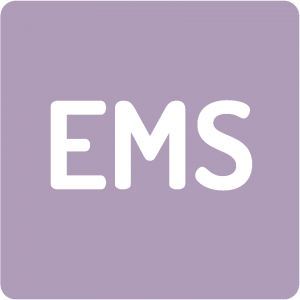# Equipe EDP, Modélisation, Simulations

##EDP, modélisation, simulation

Team animators: Boris Andreianov & Bruno Galerne

• Themes:
• Partial differential equations (PDEs) and stochastic partial differential equations (SPDEs)
• Nonlinear Elliptic and Parabolic Partial Differential Equations, Nonlocal and Integro-Differential Equations, and their Numerical Analysis [B. Andreianov, G. Barles, Y. Belaud, M.-F. Bidaut-Véron, E. Chasseigne, J. Fabbri, Ch. Georgelin, L. Véron]
• Analysis and Approximation of Conservation Laws [B. Andreianov, S. Cordier, F. James, C. Lucas, V. Perrollaz, M. Ribot, A. Sylla] : measure solutions for conservation equations, existence of solutions for coupled hyperbolic-parabolic PDEs systems, existence of solutions for quasi-geostrophic type models, well-balanced schemes for hyperbolic systems of PDEs, schemes for hyperbolic PDEs on networks, interface problems for conservation laws
• Dispersive Equations [L. Molinet, V. Perrollaz]
• Hamilton-Jacobi Equations and Deterministic Optimal Control Problems [G. Barles, E. Chasseigne, Ch. Georgelin]
• Control of Partial Differential Equations [B. Andreianov, V. Perrollaz, K.-D. Phung]
• Reaction-diffusion equations, mathematical ecology [F. Kuczma, S. Madec]
• Image processing [B. Galerne, L. Delsol, T. Haberkorn, C. Louchet, C. Lucas] : variational techniques in collaboration with probability, statistics and optimization;   clinical magnetic resonance imaging, tomography, thermo- and photo-acoustic tomography
• Kinetic equations, population dynamics and applications [J. Barré, S. Cordier, S. Mancini]
• Variational techniques
• Application areas:
• Fluid mechanics and hydrology  [S. Cordier, F. James, M. Mancini,  C. Lucas], partnership with INRA, IRD, INRIA : generalized shallow-water equations, modeling of soil erosion and sediment transport
• Biology and medicine [C. Georgelin, M. Grillot, P. Grillot, S. Madec, S. Mancini, M. Ribot] : cellular adhesion by cadherines, modeling of micro-algae biofilm for biofuel production, modeling of gut microbiota, image processing for biology, modeling the pulsatile secretion of GnRH neurons, spatial biological control of insects invasion
• Aero-space industry  [T. Haberkorn], parternship with Airbus Defense & Space and CNES : optimal control for aero-space industry
• Traffic  [B. Andreianov, A. Sylla]: macroscopic modeling of pedestrian and vehicle dynamics
• Physics [J. Barré, F. James] : phase transition models, statistical mechanics
• Economy [S. Cordier]